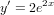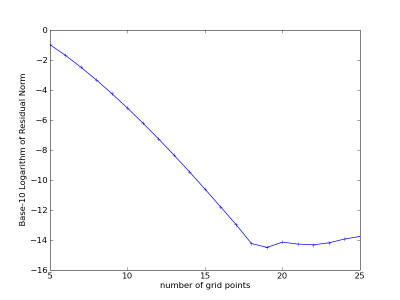## Saturday, May 30, 2009

### FFTW Discrete Cosine Transform Derivative

I've done several posts lately about finite difference methods. Spectral methods are the ultimate extension of these same basic ideas used from Boole on up to the present. For an interesting read on this progression (or lack thereof) check out the preface of Boyd's spectral methods book.

Instead of using a series of overlapping local derivative expressions, the derivative at each point is calculated with an interpolating polynomial that spans the entire grid. This leads to exponential (rather than the usual first, second, etc) order of convergence. For folks used to finite differences, Fornberg has written a concise guide that focuses especially on the connection between finite difference approximations and pseudospectral methods.

There are a couple of things that make spectral methods practical for a wide variety of engineering problems (not just periodic boundaries). The most important being Chebyshev Polynomials, which through a little change of variables can be calculated with Fourier transforms, or discrete cosine transform (DCT) for a factor of four speed-up over the FFT. Luckily the excellent FFTW library has specialized transforms for real, even data which we can use to calculate our DCTs with nice, optimized O(n*log(n)) algorithms for arbitrary length data. Here is a thread discussing the usage of FFTW's DCT for calculating derivatives.

Chebyshev polynomial in terms of cos():From the FFTW docs the forward and inverse discrete cosine transforms (DCTs) areNotice the similarity between the Chebyshev polynomial and the formula for the coefficients of the DCT.

Here's a Fortran routine that uses the DCT Type II and Type III from FFTW to calculate the derivative of a non-periodic function. An important thing to do when using spectral methods on non-periodic functions is to choose grid points that are at the roots of the Chebyshev polynomials. This makes them equally spaced in the angular coordinate, so we can use the standard transform libraries (which expect equally spaced data). Choosing nodes this way also helps with Runge's Phenomenon.

subroutine dct_diff(dydx, y, half_interval, n)
! use the discrete cosine transform from fftw to calculate the derivative
integer, intent(in) :: n ! number of data points
double precision, intent(in), dimension(n) :: y ! function values
double precision, intent(out), dimension(n) :: dydx ! derivative values
double precision, intent(in) :: half_interval ! half the interval length
double precision, dimension(n) :: beta ! derivative coefficients
double precision, dimension(n) :: alpha ! function coefficients
integer :: plan1, plan2, i
integer :: n_logical ! the logical size of the transform, size of
! the corresponding real symmetric DFT

! the logical size depends on the type of transform, check the docs:
! http://www.fftw.org/doc/1d-Real_002deven-DFTs-_0028DCTs_0029.html
n_logical = 2*(n)

! forward DCT:
call dfftw_plan_r2r_1d(plan1, n, y, alpha, FFTW_REDFT10, FFTW_ESTIMATE)
call dfftw_execute_r2r(plan1, y, alpha)
call dfftw_destroy_plan(plan1)
alpha = alpha / half_interval

! recurrence for the derivative coefficients:
beta(n) = 0D0
beta(n-1) = 2D0 * dble(n-1) * alpha(n)
do i = n-1, 2, -1
beta(i-1) = beta(i+1) + 2D0 * dble(i-1) * alpha(i)
end do
beta = - beta ! this makes it work, but not sure why!

! inverse DCT:
call dfftw_plan_r2r_1d(plan2, n, beta, dydx, FFTW_REDFT01, FFTW_ESTIMATE)
call dfftw_execute_r2r(plan2, beta, dydx)
call dfftw_destroy_plan(plan2)

! FFTW computes the un-normalized transforms, normalize by logical size
dydx = dydx / dble(n_logical)

end subroutine dct_diff

I compile this with F2Py so I can use all the nice facilities of Numpy and Scipy. Here's a Python convenience function for giving nodes based on the Chebyshev roots:

def arb_int_opt_nodes(a,b,n): # function to give Chebyshev points on an interval
y = numpy.zeros(n)
for i in xrange(n):
y[n-i-1] = numpy.cos( (2*i + 1)*numpy.pi/(2*n) )
return(((b - a) * y + b + a) / 2.0)

Try it out on a test function:with a known analytical derivative:The test function and its derivative using six grid points:Convergence graph:Pretty awesome, down to the round-off plateau with less than 20 points on the interval. This recent book on Chebyshev polynomials has a good tabulated example of integrating and differentiating a Chebyshev series on pp 33 - 35 that was very useful in debugging.

Perhaps a more practically useful test function is one-wavelength of cos(). This will give us an idea of how many nodes per wavelength we need for high accuracy solutions.Which looks to give a rule of thumb of about twenty nodes per wavelength to get down to the round-off plateau (32 bit double precision).

Scroll back up and take a look at the Fortran again, see that sign change after the recurrence? Know why it's there? Leave a comment and let me know if you think you do.

## Friday, May 22, 2009

### Maxima Functions to Generate Finite Difference Expressions

I've written a couple posts demonstrating the use of Maxima to calculate finite difference expressions of various varieties (complex step derivatives, compact differences, plain old differences). The batch files associated with those aren't very user friendly, if you want to calculate different expressions you have to go in and edit them in several places. So I decided to write a couple functions that would take two arguments and return the finite difference expression you asked for.

Maxima file for explicit finite difference expressions.

Maxima file for implicit or compact finite difference expressions.

Usage is just:

foo : explicit_fde([-1,0,1],1);

which would put a central difference expression for the first derivative into foo. The first argument is a list representing the stencil to use, the second argument is the derivative you want to estimate.

The implicit expressions are similar:

foo : implicit_fde([-1,0,1],1,1);

The extra argument is so that you don't get the trivial solution back (see this post for details). Also, the return value for the implicit expression is the augmented coefficient matrix for the first derivative. That means the last column is the explicit side of the expression (terms involving the function value), and the first n columns are the coefficients for the first derivative at the stencil points.

Thanks to the helpful folks on the Maxima List for fixing some of my mistakes and giving me some good debugging tips.

## Wednesday, May 6, 2009

### Open Math Tools Custom Search Engine

I found a really simple how-to for creating custom Google search engines and used it to make my own little Open Source Math Tools search engine. I find it really annoying when I'm searching for documentation or examples in Maxima that the results are full of Nissan Maxima pages. Things are even worse when trying to search for stuff relevant to R.

Using the custom search engine I don't have to specify that I'm searching for Maxima, Octave, R or SciPy stuff, because I already told Google to favor results from those pages. Right now the custom search engine (CSE) only has about twenty sites included here's the big ones (list updated 10 Nov 2009):

And of course the mailing list archives for those projects as well. The idea of this CSE is to help the user find articles, tutorials and documentation about using open source math tools to do specific tasks.

The results are pretty good. I choose the option of including broader web search results, so high ranking results from Wikipedia or MathWorld still show up, but the first page is full of relevant links to the favoured sites. For instance the results for 'bayes model averaging' have a mix of about half and half papers from academic websites and links to various R project pages, or the results for the Maxima function augcoefmatrix() which links to the Maxima manual as well as several web sites with usage examples.

It really improves the signal to noise ratio. Leave a comment if there's a site you think I'm missing.

## Friday, May 1, 2009

### Deriving Compact Differences with Maxima

I wrote a while back about using Maxima to derive asymmetric complex step finite difference expressions for derivatives. The basic approach was sound, but it didn't really use much of the power of the built-in symbolic computation capability. This post will explore deriving compact difference relations with Maxima and avoid all the for-loops by using Maxima's built-in knowledge of Taylor series and Linear Algebra.

First we'll use the taylor() function to define truncated Taylor series expressions for the function and it's first derivative. Then we'll us the ev() function to evaluate the expressions with different values of x: x0, x0+h, and x0-h. Where h is the step size.

f_taylor : taylor(f(x),x,x0,5);
fp_taylor : taylor('diff(f(x),x),x,x0,4);

rhs_list : [at(f(x),x=x0),
'at('diff(f(x),x), x=x0),
'at('diff(f(x),x,2), x=x0),
'at('diff(f(x),x,3), x=x0),
'at('diff(f(x),x,4), x=x0),
'at('diff(f(x),x,5), x=x0)
];

f_coefs : submatrix(factor(augcoefmatrix([f_taylor], rhs_list)), 7);
fp_coefs : submatrix(factor(augcoefmatrix([fp_taylor], rhs_list)), 7);

Using the augcoefmatrix() function is an easy way to extract the coefficients of the various derivatives in the Taylor series. Coefficients of the function expansion:Coefficients in the first derivative expansion:Now we need to pack the coefficients for each point into a matrix.

/* add the columns for the points with function values: */

/* add the columns for the points with function derivative values: */

The matrix A represents the linear system we need to solve to find the coefficients of the function value and its derivative to use in our finite difference approximation.Since we might want to solve for more than one expression, it's a good idea to factor A rather than just inverting it. The matrix should look familiar if you've used a Taylor Table before.

/* we need to invert A to get finite difference formulas so factor it: */
M : lu_factor(A);
[P,L,U] : get_lu_factors(M);Now it is a straight forward matter to generate a right-hand-side vector for our linear system and solve for the unknown coefficients. The numerical rhs-vector represents the derivatives of the function.Putting a one in the appropriate position of the vector gives the corresponding finite difference expression for that derivative.

/* right hand side vector for getting the first derivative: */
b_fp : matrix(,,,,,);
/* coefficients for the compact difference formula: */
a_fp : fullratsimp(lu_backsub(M, b_fp));
/* values vector (function and derivative at the points): */
vv : matrix([at(f(x),x=x0-h),
at(f(x),x=x0),
at(f(x),x=x0+h),
'at('diff(f(x),x),x=x0-h),
'at('diff(f(x),x),x=x0),
'at('diff(f(x),x),x=x0+h)
]);
/* compact difference formula for the first derivative: */
cd_fp : vv.a_fp;
cd_fp_reduced : expand((h**4) * (cd_fp) / 30);

You might notice the extra 1 in the 6th place of the right-hand-side vector, it's there so we don't get the trivial solution of using the value for the derivative at x=x0 for our estimate of the value of the derivative (we obviously don't know it yet). This results in the following difference expression.Which can be multiplied by an arbitrary constant to achieve a more computation ready form.The h**4 term can be neglected, resulting in the well-known fourth order compact (implicit) expression for the first derivative.

Here's the Maxima batch file used for this.